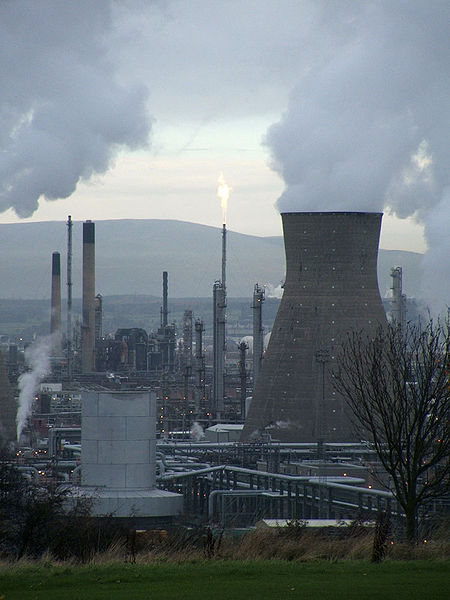eBook – Economic importance of trees

# How to calculate CO2 emissions from fossil fuel combustion?Fossil fuels are GHG emission intensive and release GHG’s when combusted. Growing concern towards climate change and the contribution of anthropogenic activities towards it, calculating carbon emissions from combustion of fossil fuels is very necessary. Nowadays many organizations have started creating GHG inventory of their day to day activities.

Following guidelines will help you to calculate carbon dioxide emission from the burning of fossil fuels.

1. Collect data on the quantity of fuel combusted on a volume, mass, or energy basis. These data can be based on fuel receipts, purchase records, or metering of the amount of fuel entering the combustion device.
2. Then collect data on the fuel’s density and/or calorific/heating value and convert fuel data to a common volume, mass, or energy content basis. The fuel consumption data and the carbon content data should be in a common unit before calculating emissions.
3. Estimate carbon content of each of the fuels combusted. Carbon content values can be based on laboratory fuel analysis, data provided by fuel suppliers, or default factors (IPCC).
4. Collect data to determine oxidation fraction. You can use default factors (IPCC).

Note: Check to ensure that all units are consistent and converted correctly across parameters and facilities.

The below mentioned fossil fuels are used in mobile and stationary equipments. The formula for developing carbon emission factor for the fossil fuels is given below:

Emission factor (Kg CO2/unit) = {Default carbon content * Oxidation factor * Default Net calorific value * Carbon molecule mass ratio (44/12) * Fuel Density}

 Fuel (Litres / kg) Default carbon content (Kg/GJ) Oxidation factor Net calorific value (TJ/Gg) Carbon molecule mass ratio Fuel density (Kg/Litre) Carbon emission factor (Kg/Litre) Diesel 20.2 1 43 44/12 0.845 2.691 Petrol 18.9 1 44.3 44/12 0.775 2.379

You can covert final value (Carbon dioxide in kg/Litre) into tCO2 equivalent by dividing the kg unit by 1000.

The references for various values taken in the table above are;

1. Default carbon content in each of the fuel came from the table 1.3, Chapter 1, Volume 2 of IPCC Guidelines for National GHG Inventories 2006.
2. Default oxidation factor in each of the fuel came from the table 1.4, Chapter 1, Volume 2 of IPCC Guidelines for National GHG Inventories 2006.
3. Default net calorific value in each of the fuel came from the table 1.2, Chapter 1, Volume 2 of IPCC Guidelines for National GHG Inventories 2006.

Another Method (As described in CDM tool);

CO2 emissions from fossil fuel combustion are calculated based on the quantity of fuels combusted and the CO2 emission coefficient of those fuels, as follows;

 CO2 emissions from fossil fuel combustion (tCO2) = Quantity of fuel combusted (mass or volume unit) X COEF (CO2 emission coefficient of fuel in tCO2/mass or volume unit)

The CO2 emission coefficient (COEF) can be calculated using one of the following two Options, depending on the availability of data on the type of fossil fuel as follows:

1. The CO2 emission coefficient COEFis calculated based on the chemical composition of the fossil fuel   using the following approach;

If the quantity of fuel combusted is in mass unit;

 COEF = Weighted average mass fraction of carbon in type of fuel (tC/mass unit of the fuel) X 44/12

If the quantity of fuel combusted is in a volume unit;

 COEF = Weighted average mass fraction of carbon in type of fuel (tC/mass unit of the fuel) X Weighted average density of type of fuel (Mass unit/volume unit of the fuel) X 44/12

2.   The CO2 emission coefficient COEFis calculated based on net calorific value and CO2 emission factor of the type of fuel, as follows;

 COEF = Weighted average net calorific value(NCV) of the fuel  (In GJ/mass or volume unit) X Weighted average CO2 emission factor of the type of fuel  (In tCO2/GJ)

By using both these calculation methodologies, you can calculate CO2 emissions from fossil fuel combustion. Use any one relevant to the requirement.

Sources: UNFCCC CDM website, GHG Protocol and IPCC

Know more about Global Warming Potential of different Green House Gases.

1.2.3.•4.•